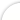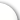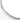Банк рефератов содержит более 364 тысяч рефератов, курсовых и дипломных работ, шпаргалок и докладов по различным дисциплинам: истории, психологии, экономике, менеджменту, философии, праву, экологии. А также изложения, сочинения по литературе, отчеты по практике, топики по английскому.Полнотекстовый поиск.ya-page_js_yes .ya-site-form_inited_no { display: none; }Всего работ: 364139Теги названийРазделыАвиация и космонавтика (304)Административное право (123)Арбитражный процесс (23)Архитектура (113)Астрология (4)Астрономия (4814)Банковское дело (5227)Безопасность жизнедеятельности (2616)Биографии (3423)Биология (4214)Биология и химия (1518)Биржевое дело (68)Ботаника и сельское хоз-во (2836)Бухгалтерский учет и аудит (8269)Валютные отношения (50)Ветеринария (50)Военная кафедра (762)ГДЗ (2)География (5275)Геодезия (30)Геология (1222)Геополитика (43)Государство и право (20403)Гражданское право и процесс (465)Делопроизводство (19)Деньги и кредит (108)ЕГЭ (173)Естествознание (96)Журналистика (899)ЗНО (54)Зоология (34)Издательское дело и полиграфия (476)Инвестиции (106)Иностранный язык (62791)Информатика (3562)Информатика, программирование (6444)Исторические личности (2165)История (21319)История техники (766)Кибернетика (64)Коммуникации и связь (3145)Компьютерные науки (60)Косметология (17)Краеведение и этнография (588)Краткое содержание произведений (1000)Криминалистика (106)Криминология (48)Криптология (3)Кулинария (1167)Культура и искусство (8485)Культурология (537)Литература : зарубежная (2044)Литература и русский язык (11657)Логика (532)Логистика (21)Маркетинг (7985)Математика (3721)Медицина, здоровье (10549)Медицинские науки (88)Международное публичное право (58)Международное частное право (36)Международные отношения (2257)Менеджмент (12491)Металлургия (91)Москвоведение (797)Музыка (1338)Муниципальное право (24)Налоги, налогообложение (214)Наука и техника (1141)Начертательная геометрия (3)Оккультизм и уфология (8)Остальные рефераты (21692)Педагогика (7850)Политология (3801)Право (682)Право, юриспруденция (2881)Предпринимательство (475)Прикладные науки (1)Промышленность, производство (7100)Психология (8692)психология, педагогика (4121)Радиоэлектроника (443)Реклама (952)Религия и мифология (2967)Риторика (23)Сексология (748)Социология (4876)Статистика (95)Страхование (107)Строительные науки (7)Строительство (2004)Схемотехника (15)Таможенная система (663)Теория государства и права (240)Теория организации (39)Теплотехника (25)Технология (624)Товароведение (16)Транспорт (2652)Трудовое право (136)Туризм (90)Уголовное право и процесс (406)Управление (95)Управленческие науки (24)Физика (3462)Физкультура и спорт (4482)Философия (7216)Финансовые науки (4592)Финансы (5386)Фотография (3)Химия (2244)Хозяйственное право (23)Цифровые устройства (29)Экологическое право (35)Экология (4517)Экономика (20644)Экономико-математическое моделирование (666)Экономическая география (119)Экономическая теория (2573)Этика (889)Юриспруденция (288)Языковедение (148)Языкознание, филология (1140)# Реферат: Optimal Spacecraft Trajectories Essay Research Paper Optimal

 Название: Optimal Spacecraft Trajectories Essay Research Paper Optimal Раздел: Топики по английскому языку Тип: реферат Добавлен 07:59:14 25 октября 2010 Похожие работы Просмотров: 7 Комментариев: 13 Оценило: 2 человек Средний балл: 5 Оценка: неизвестно     Скачать Optimal Spacecraft Trajectories Essay, Research Paper Optimal Finite-Thrust Spacecraft Trajectories Using Collocation and Nonlinear Programming Background Recently there has been great interest by NASA and the ESA in developing satellite constellations that will serve to act as interferometers for use in gravity wave detection. These satellite constellations would be composed of six satellites arranged in varying orbits such that they form an equilateral triangle with each triangle vertex containing two satellites at close proximity. Each vertex would send two laser signals to the satellites at the other vertices. The satellites then calculate the distance between themselves and the other vertices based on the time it took the laser beam to reach them. This distance is compared with the known distance between the satellites and any increase would suggest that a gravity wave has passed through the detector. The job of the astrodynamicist is to keep the satellites in their orbits so that the distance between each vertex remains fixed. This starts off as a problem of forced-Keplerian motion but then gets a little more complicated when the constraint of minimizing thruster firing time is thrown in. When the satellite thrusters are firing to maintain their orbit, the interferometer can’t take accurate data because the disturbance due to the movement is far greater than the disturbances caused by gravity waves. This dilemma brings about the problem of needing to minimize the thruster firing time so that you can maximize the data collection time. One method of optimizing finite-thrust spacecraft trajectories is the Direct Collocation with Nonlinear Programming approach (DCNLP). Direct Collocation with Nonlinear Programming Direct Collocation with Nonlinear Programming is a method of approximating the continuous optimization problem with a parameter optimization problem. This method has been developed over the course of the last thirty-five years and a summary of its history can be found in reference 1. The DCNLP approach approximates the spacecraft trajectory with piecewise polynomials that are represented by state and control variables at a given number of nodes. State variables are stored in the state vector x and the control variables are stored in the control vector u . The spacecraft trajectory is divided into a series of thrust arcs and coast arcs, which are subdivided into evenly spaced segments. In a segment each state variable has a state trajectory which is taken to be a Hermite cubic. This is a “unique cubic that goes through the endpoints of the thrust segment with the appropriate derivatives that are dictated by the evaluation of the differential equations of motion at the endpoints” . Each control variable is assumed to have a linear control trajectory across the segment. At the center of each segment a collocation point is found and then the difference between the derivative of the Hermite cubic at the collocation point and the trajectory equations of motion evaluated at the collocation point is calculated. This difference is called the defect and it is one of the constraints that the objective function, the thruster firing time, is subject to. When the defect is zero the differential equations of motion are satisfied at the collocation point and the endpoints. Allowing the trajectory equations of motion to be given by = (x,u) and the length of time of a segment to be T, the Hermite interpolated state vector at the collocation point is given by xc = (1/2)+(xl + xr) + (T/8)+[ (xl, ul) - (xr, ur)] where xl and xr are the state vectors at the left and right nodes. The linearly interpolated control vector is given by uc = (1/2)+(ul + ur) where ul and ur are the control vectors at the left and right nodes. The derivative of the Hermite interpolated state vector at the collocation point is c = -[3/(2+T)]+(xl – xr) – (1/4)+ [ (xl, ul) + (xr, ur)] Finally, the defect vector is d = (xc,uc) – c where both the equations of motion and the derivative of the Hermite interpolated state vector are evaluated at the collocation point ,,,. The differential equations of motion that describe the spacecraft trajectory are needed for use in the equations above. The three-dimensional equations of motion for a thrust arc in spherical coordinates using canonical units (m = 1.0) are where c1 is the orbital radius, c2 is the angle between the inertial Z-direction and the satellite radius vector, c3 is the radial component of velocity, c4 is the c2 component of velocity, c5 is the angle between the inertial X-direction and the satellite radius vector, c6 is the c5 component of velocity, at is the magnitude of the thrust acceleration, u1 is the c2 component of the thrust acceleration, u2 is the c5 component of the thrust acceleration, Fd,r is the radial component of the drag force, Fd,f is the c2 component of the drag force, and Fd,q is the c5 component of the drag force. The equations of motion for a coast arc will be identical to those of a thrust arc except that the thrust acceleration terms specified by u1, u1, and at will be zero. Besides the defect vector, equality and inequality constraints can also be added to the analysis. These are determined by the user and can include for example, constraints on the initial or final conditions or path constraints. All of the defects and constraints are used as bounds on the objective function, the quantity to be minimized. In the case of the spacecraft trajectories, the objective function is the sum of the thrust arc durations. The state, control, and defect variables for both thrust and coast arcs plus any other problem constraints make up a nonlinear programming problem. To solve this problem the variables must first be assembled into state and constraint vectors. The state and control vectors and the thrust arc duration time are assembled into the nonlinear programming ( NLP) state vector as follows XT = [ x1T, u1T, x2T, u2T, , xnT, unT, t1, t2, , tk] where X is the NLP state vector, n is the number of nodes that the trajectory is divided into, t is the thrust arc duration time, and k is the number of thrust arcs on the trajectory. The defects, equality constraints, and inequality constraints are assembled into the NLP constraint vector as follows CT = [ dt,1, dc,1, dt,2, dc,2, , dt,m, dc,p, h1, h2, , hj, g1, g2, , gi] where C is the NLP constraint vector, m is the number thrust arc segments, p is the number of coast arcs, h is the equality constraints, j is the number of equality constraints, g is the inequality constraints, and i is the number of inequality constraints ,,,. The NLP state vector must always be larger in length than the NLP constraint vector or an optimized solution will not be found. Once the NLP state and constraint vectors are found, a solution can be computed by placing these vectors into an optimization subroutine such as NPSOL, MINOS, or SQP. References  Enright, P.J., and Conway, B.A., “Optimal Finite-Thrust Spacecraft Trajectories Using Collocation and Nonlinear Programming,” Journal of Guidance, Control, and Dynamics, Vol. 14, No. 5, September-October 1991, pp. 981-985.  Enright, P.J., and Conway, B.A., “Discrete Approximations to Optimal Trajectories Using Direct Transcription and Nonlinear Programming,” Journal of Guidance, Control, and Dynamics, Vol. 15, No. 4, July-August 1992, pp. 994-1002.  Betts, J.T., and Huffman, W.P., “Application of Sparse Nonlinear Programming to Trajectory Optimization,” Journal of Guidance, Control, and Dynamics, Vol. 15, No. 1, January-February 1992, pp. 198-206.  Betts, J.T., and Huffman, W.P., “Path-Constrained Trajectory Optimization Using Sparse Sequential Quadratic Programming,” Journal of Guidance, Control, and Dynamics, Vol. 16, No. 1, January-February 1993, pp. 59-68.
Оценить/Добавить комментарий
Имя
Оценка
Комментарии:
Привет студентам) если возникают трудности с любой работой (от реферата и контрольных до диплома), можете обратиться на FAST-REFERAT.RU , я там обычно заказываю, все качественно и в срок) в любом случае попробуйте, за спрос денег не берут)
 Olya 23:29:38 28 августа 2019
.
 . 23:29:37 28 августа 2019
.
 . 23:29:37 28 августа 2019
.
 . 23:29:36 28 августа 2019
.
 . 23:29:35 28 августа 2019

Смотреть все комментарии (13)
Работы, похожие на Реферат: Optimal Spacecraft Trajectories Essay Research Paper Optimal

НазадМенюГлавнаяРефератыБлагодарностиОпросСтанете ли вы заказывать работу за деньги, если не найдете ее в Интернете?

 Да, в любом случае. Да, но только в случае крайней необходимости. Возможно, в зависимости от цены. Нет, напишу его сам. Нет, забью.

Результаты(258412)
Комментарии (3474)Copyright © 2005-2020 BestReferat.ru support@bestreferat.ru реклама на сайте